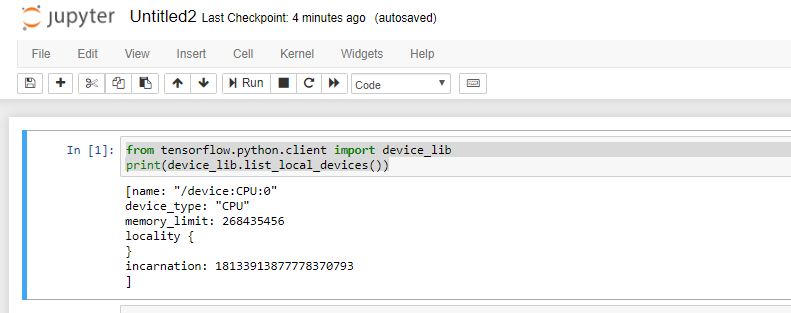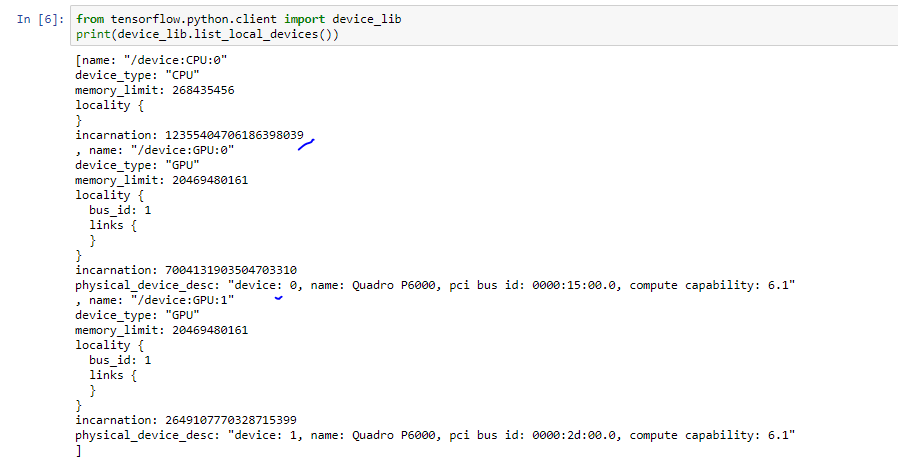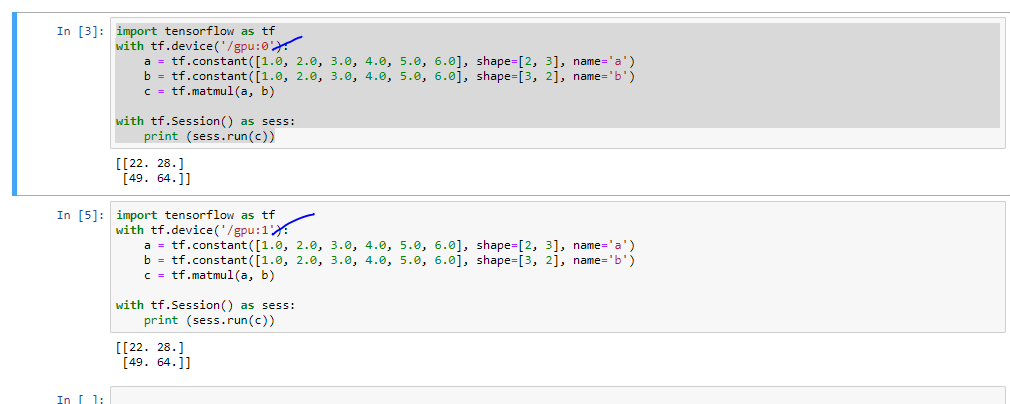# Python – Check TensorFlow Using GPU

By | July 10, 2019

To check weather Tensorflow using GPU follow these steps:

```from tensorflow.python.client import device_lib
print(device_lib.list_local_devices())```

If GPU support is not enabled you will see the output as followsIf you have GPU support enabled then you will see the output as follows.Run Below code to assign specific GPU to your code if you have multiple GPU

```import tensorflow as tf
with tf.device('/gpu:0'):
a = tf.constant([1.0, 2.0, 3.0, 4.0, 5.0, 6.0], shape=[2, 3], name='a')
b = tf.constant([1.0, 2.0, 3.0, 4.0, 5.0, 6.0], shape=[3, 2], name='b')
c = tf.matmul(a, b)

with tf.Session() as sess:
print (sess.run(c))

```